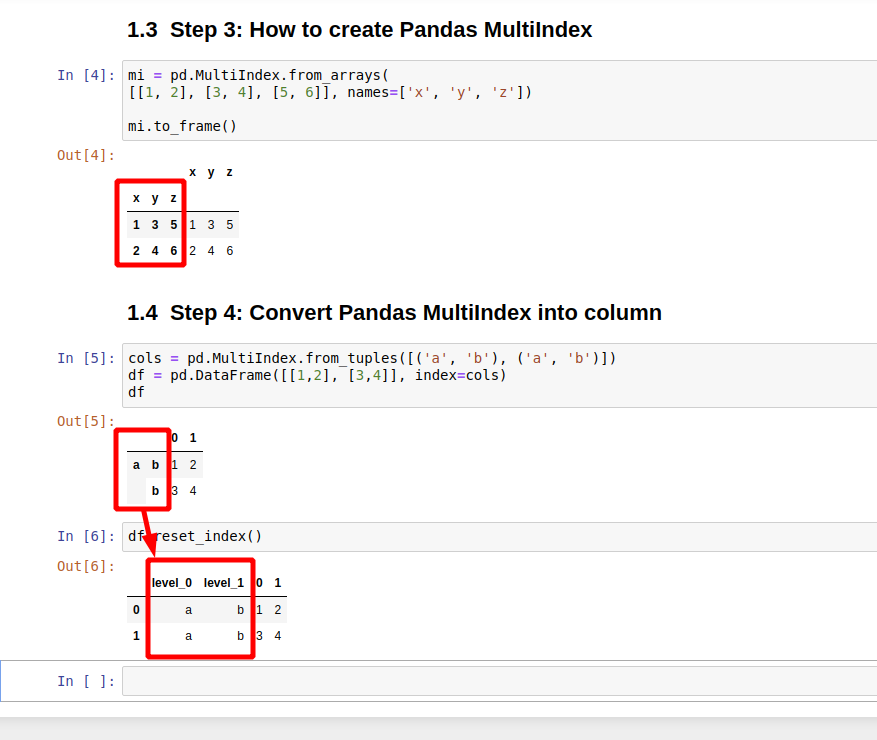In Pandas MultiIndex is advanced indexing techniques for DataFrames. It allows multiple levels for the indexes. It can be called also - hierarchical index or multi-level index.

In Pandas indexes are represented as a labeled axis stored as an object. They help for:

• identify data
• data alignment
• get and set of subsets

## Step 1: DataFrame MultiIndex in Pandas

Let's create a MultiIndex DataFrame in Pandas. The first example shows MultiIndex for the rows:

``````cols = pd.MultiIndex.from_tuples([(0, 1), (0, 1)])
pd.DataFrame([[1,2], [3,4]], index=cols)
``````

which will produce DataFrame with two level index:

• 0 is the level 0
• 1 is the level 1

Note: In this case 0 and 1 are the same in the levels which are not mandatory.

0 1
0 1 1 2
1 3 4

The next example will create a hierarchical index for the columns. As you can see there are two levels of the column index:

• a - which is level - 0
• b - is considered as level 1
``````cols = pd.MultiIndex.from_tuples([('a', 'b'), ('a', 'b')])
pd.DataFrame([[1,2], [3,4]], columns=cols)
``````
a
b b
0 1 2
1 3 4

## Step 2: Pandas MultiIndex to single index

In this step will check how to convert a multi-level index to a single level one. This can be done by dropping levels from the MultiIndex:

``````df.droplevel(level=0, axis=1)
``````

which will result in:

b b
0 1 2
1 3 4

and

``````df.droplevel(level=1, axis=1)
``````

which will result in:

a a
0 1 2
1 3 4

Note 1: Rows or column can be selected by parameter - `axis`
Note 2: If you try to use the labelled value: 'a' or 'b' like:

`df.droplevel(level='b', axis=1)` you will get an error:

## Step 3: How to create Pandas MultiIndex

There are many ways to create a DataFrame with Pandas MultiIndex. We already saw the one which uses the method: `from_tuples`.

Now let's check another one - `from_arrays`:

``````mi = pd.MultiIndex.from_arrays(
[[1, 2], [3, 4], [5, 6]], names=['x', 'y', 'z'])

mi.to_frame()
``````

which will result in:

x y z
x y z
1 3 5 1 3 5
2 4 6 2 4 6

## Step 4: Convert Pandas MultiIndex into column

Another useful operation for MultiIndex DataFrames is to convert levels into columns. This can be done in several ways:

• `stack`/`unstack`
• `pivot`
• `crosstabs`
• `reset_index`

Let's check an example for: `reset_index`. So let's have the next DataFrame:

``````cols = pd.MultiIndex.from_tuples([('a', 'b'), ('a', 'b')])
df = pd.DataFrame([[1,2], [3,4]], index=cols)
``````
0 1
a b 1 2
b 3 4

To convert MultiIndex to columns or rows can be done by:

``````df.reset_index()
``````

which will output:

level_0 level_1 0 1
0 a b 1 2
1 a b 3 4